Sponsored Link •Weblogs Forum
The Adventures of a Pythonista in Schemeland/18

0 replies on 1 page.

 Welcome Guest   Sign In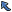Back to Topic List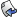Reply to this Topic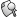Search Forum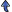Threaded View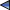Previous Topic Next Topic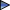Flat View: This topic has 0 replies on 1 page
 Michele Simionato Posts: 222 Nickname: micheles Registered: Jun, 2008
The Adventures of a Pythonista in Schemeland/18 (View in Weblogs)
Posted: Mar 9, 2009 1:29 AMReply
Summary
This episode is about streams, a typical data structure of functional languages. The differences between functional streams and imperative iterators are discussed. En passant, I give a solution of the classic eight queens problem.
 Advertisement# Streams

This episode is about streams, a typical data structure of functional languages. The differences between functional streams and imperative iterators are discussed. En passant, I give a solution of the classic eight queens problem.

# The eight queens puzzle

Before starting the analysis of streams, I want to close the discussion about list comprehension. Last week I had no time to discuss one of the conveniences of the list-of macro, i.e. the ability to define internal variables with a (name is value) syntax. To give an example of that, I have decided to show you a solution of the infamous eight-queens puzzle that you will find in all the theoretical textbooks about programming.

In my opinion the eight queens puzzle is not so interesting, however, if you want to study Scheme, you will find this kind of academical examples everywhere, so I made my concession to the tradition. In particular, the official document about streams in R6RS Scheme, i.e. SRFI-41, contains a solution of the eight queens puzzle by using the same algorithm I am presenting here, but with streams instead of lists. You may want to compare the list solution to the stream solution.Animation taken from Wikipedia

The algorithm is based on a clever trick which is quite common in the mathematical sciences: to introduce an additional degrees of freedom which apparently makes the problem harder, but actually gives us a fast lane towards the solution. Here the clever idea is to change the question and to considered not a single square chessboard, but a family of rectangular chessboards with n rows and N columns (with n<=N and N=8). Of course we are interested in the n=8 solution; however, keeping n generic helps, since an easy solution can be found for small n (in particular for n=1) and we can figure out a recursive algorithm to build the n+1 solution starting from the n=1 solution, until we reach n=8.

Let us express a solution as a list of column positions for the queens, indexed by row. We will enumerate rows and columns starting from zero, as usual. The case n=1 (putting a queen on a 1x8 chessboard) has 8 solutions, expressible as the list of lists '((0)(1)(2)(3)(4)(5)(6)(7)) - the first (and only) queen will be at row 0 and columns 0, 1, 2, 3, 4, 5, 6 or 7. If there are two queens (n=2) one has more solutions; for instance the first queen (i.e. the one at row 0) could be at column 0 and the second queen (i.e. the one at row 1) at column 2, and a solution is (0 2). The solutions for the n-queens problem are found by looking at the possible new configurations, starting from the solutions of the n-1-queens problem and by discarding the forbidden ones.

A configuration is forbidden if two queens are on the same column (by construction they cannot be in the same row, since they are indexed by row) or on the same diagonal. The condition being on the same diagonal translates into the difference between the row coordinates is the same as the difference between the column coordinates, in absolute value. Here is the condition expressed in Scheme and making use of list-of and of the is syntax, where new-row and new-col is the tentative position of the n-th queen and safe-config is a solution of the n-1-queen problem:

```(define (all-true lst) ;; a Python-like all, true if all elements are true
(for-all (lambda (x) x) lst))

(define (safe-queen? new-row new-col safe-config)
;; same-col? and same-diag? are boolean inner variables
(all-true (list-of (not (or same-col? same-diag?))
((row col) in (enumerate safe-config))
(same-col? is (= col new-col))
(same-diag? is (= (abs (- col new-col)) (abs (- row new-row))))
)))
```

safe-queen? checks that the new configuration is safe by looking at all the queens already placed. We can find all the solutions with a recursive function:

```(define (queens n N)
(if (zero? n) '(())
(list-of (append safe-config (list col))
(n-1 is (- n 1)); inner variable
(safe-config in (queens n-1 N))
(col in (range N))
(safe-queen? n-1 col safe-config)
)))
```

In particular we can check that the n=8 problem has 92 solutions:

```> (length (queens 8 8))
92
```

I refer you to Wikipedia for nice drawings of the solutions.

# Iterators and streams

Python programmers are well acquainted with generators and iterators, and they know everything about lazyness. In particular they know that the Python iterator

```>>> it123 = iter(range(1, 4))
```

is left unevaluated until its elements are requested. However, the Python way is only superficially similar to the truly functional way, found in Haskell or in Scheme. Actually, when Python copies from functional languages, it does so in an imperative way. Here the iterator it123 is an object with an internal state; there is a next method which allows to change the state. In particular, if you call .next() twice, the same iterator returns different values:

```>>> it123.next()
1
>>> it123.next()
2
```

Thus, Python iterators are not functional. Functional languages such as Scheme, ML and Haskell have no imperative iterators: they have streams instead. Ikarus comes with a built-in stream library, so that I can give a concrete example right now (of course you can use streams in other implementations simply by using the reference implementation described in SRFI-41). Here is how to define a stream on the numbers 1,2,3:

```> (import (streams))
> (define str123 (stream-range 1 4))
```

There is no equivalent of the next method for streams, since there is no concept of internal state of a stream. However, there is a stream-car procedure which takes the first element of a stream, and a stream-cdr procedure returning another stream which lacks the first element. Both procedures are functional, i.e. they act without mutating the original stream object in any way. In particular, if you apply stream-car twice, you get always the same result:

```> (stream-car str123)
1
> (stream-car str123)
1
```

In Python, looping on an iterator exhausts it, and running twice the same loop can have unexpected results:

```>>> chars = iter('abc')
>>> for c in chars: print c,
...
a b c
>>> for c in chars: print c,
...
```

The first time the loop prints "a b c", but the second time it does not print anything. In a functional language the same code must have the same effect, it cannot depend from the inner state of the stream. Actually, this is what happens:

```> (define chars (stream #\a #\b #\c))
> (stream-for-each display chars)
abc> (stream-for-each display chars)
abc>
```

stream-for-each is an utility from SRFI-41, with obvious meaning. Actually SRFI-41 offers a series of convenient features. The most useful one is stream comprehension, which is very similar to list comprehension. Since I copied the list comprehensions syntax from the work of Phil Bewig, which is the author of the stream library, it is not surprising that the syntax looks the same. The difference between list comprehensions and stream comprehension is that stream comprehension is lazy and can be infinite. This is similar to Python generator expressions (genexps). For instance, in Python we can express the infinite set of the even number as a genexp

```>>> import itertools
>>> even = (i for i in itertools.count(0) if i % 2 == 0)
```

whereas in Scheme we can express it as a stream:

```> (define even (stream-of i (i in (stream-from 0)) (zero? (modulo i 2))))
```

However the Scheme stream is immutable, whereas the Python genexp is not. It is possible to loop over a stream with stream-for-each, stream-map and stream-fold; such higher order functions work as they counterparts for lists, but they return streams. There is also a stream-let syntax, which is stream version of named let, useful when applying the accumulator pattern to streams, and a function stream->list with does the obvious.

I am not explaining all the fine details, since the documentations of the SRFI is pretty good and exhaustive. As I anticipated, there is also a solution of the eight queen problem using streams that you may look at. The difference between the stream solution and the list comprehension solution is that the first one is lazy, i.e. you get one solution at the time, on demand, whereas the second one is eager: it computes all the solutions in a single bunch, and returns them together.

# Lazyness is a virtue

The basic feature of streams, apart from immutability, is true lazyness. Streams are truly lazy since they perform work only when forced - i.e. only when an element is explicitly requested - and even there if they had already accomplished a task they do not perform it again - i.e. they memoize the elements already computed (and this is not the case for Python iterators). An example should make these two points clear. Let us define a work procedure which protests when called:

```> (define (work i)
(display "Life is hard!\n") i)
```

The protest is expressed an a side effect; other than that, the function, does not perform too much, since it just returns the parameter got in input, but, you know, there is no limit to lazyness!

Now let me define a stream which invokes the function work three times:

```> (define work-work-work (stream-of (work i) (i in (stream-range 1 4))))
```

Since the stream is lazy, it does not perform any work at definition time. It starts working only when elements are expressly required:

```> (stream->list work-work-work)
Life is hard!
Life is hard!
Life is hard!
(1 2 3)
```

Now the values 1, 2, 3 have been memoized: if we try to loop again on the stream, it will return the precomputed values:

```> (stream->list work-work-work)
(1 2 3)
```

This shows clearly that the function work is not called twice. It is also clear that, had work some useful side effect (such as writing a log message) then using a stream would not be a good idea, since you could loose some message. Streams are a functional data structure and it is good practice to use only pure functions with them, i.e. functions without side effects. Moreover, I should also notice that the memoization property implies that a stream can take an unbound amount of memory, whereas an imperative iterator has no such issue.

I could say more. In particular, there are lots of caveats about streams, which are explained in detail in the SRFI-41 documentation (so many caveats that I personally do not feel confident with streams). I am also sure that Pythonistas would be even more interested in true generator-expressions and generators, which can be definite in Scheme by using continuations. However, investigating that direction will astray us away from our path. The intention of this third cycle of Adventures was just to give a feeling of what does it mean to be a true functional language, versus being an imperative language with a few functional-looking constructs.

With this episode this cycle of our Adventures ends, but a new one will begin shortly. Stay tuned!Previous Topic Next TopicSponsored LinksWeb Artima.com

Copyright © 1996-2018 Artima, Inc. All Rights Reserved. - Privacy Policy - Terms of Use